# 5 Types of Inheritance in C++ | Detail Explained with Program

### What is Inheritance in C++?

It is the technique of deriving a new class from the class which already exists.

The new class is called derived class and the old class is called the base class.

You will understand more practically while going through this tutorial.

• It is one of the prominent features offered by object-oriented programming which increases the reusability of the code.
• Reusing existing member functions reduces the lines of code.

Syntax:

```class Derived : access_modifier Base
{
Body of class Derived;
}```

The colon (:) indicates that the class Derived is derived from the class Base.

And the access modifier is either public or private.

When it is public then the public and protected data members of Base become public members of Derived.

And when the access modifier is private, then the public and protected data members of Base become private members of Derived.

### Different Types of Inheritance in C++

On broadly classifying, there are 5 major types of inheritance.

#### 1. Single Inheritance:

In this, only one class is derived from one base class.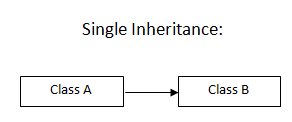C++ Program for Single Inheritance:

```#include <iostream>
using namespace std;
class A
{
public:
int a,b;
void getdata()
{
cout<<"Enter two numbers: "<<endl;
cin>>a>>b;
}
};
class B:public A
{
int c;
public:
void showdata()
{
c=a*b;
cout<<"The entered numbers are: "<<a<<" and "<<b<<endl;
cout<<"The product is: "<<c<<endl;
}
};
int main()
{
B obj;
obj.getdata();
obj.showdata();
return 0;
}
```

Output:

```Enter two numbers: 22 20
The entered number are 20 and 22.
The product is 440```

Here,

• Class B is inhering class A to access the function getdata().
• Object obj is the object of class B and it is calling inherited function getdata() of class A.

#### 2. Multilevel Inheritance:

It is an extended version of single inheritance, where other classes are further derived from the derived class.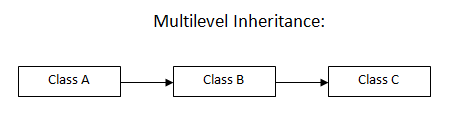C++ Program for Multilevel Inheritance:

```#include <iostream>
using namespace std;
class A
{
public:
int a,b;
void getdata()
{
cout<<"Enter two numbers: "<<endl;
cin>>a>>b;
}
};
class B:public A
{
public:
int c;
void avgdata()
{
c=(a*b)/2;
}
};
class C:public B
{
public:
void showdata()
{
cout<<"The entered numbers are "<<a<<" and "<<b<<endl;
cout<<"Their average is  "<<c<<endl;
}
};
int main()
{
C obj;
obj.getdata();
obj.avgdata();
obj.showdata();
return 0;
}
```

Output:

```Enter two numbers: 12 24
The entered numbers are 12 and 24
Their average is 18.```

#### 3. Multiple Inheritance:

In this, a derived class is invoked from more than one base class.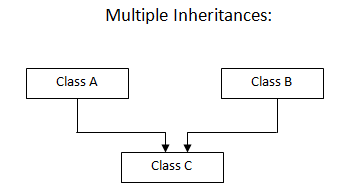C++ Program for Multiple Inheritance:

```</pre>
#include <iostream>
using namespace std;
class A
{
public:
int i;
void geti()
{
cout<<"Enter the number: "<<endl;
cin>>i;
}
};

class B
{
public:
int j;
void evaluate()
{
if(j%2==0)
{
cout<<"Entered number is even."<<endl;
}
else
{
cout<<"Entered number is odd."<<endl;
}
}
};

class C : public A, public B
{
public:
void display()
{
cout<<"Multiple inheritance successfully executed."<<endl;
}
};

int main()
{
C obj;
obj.geti();
obj. j=obj.i;
obj.evaluate();
obj.display();
return 0;
}
```

Output:

```Enter the number: 76
Entered number is even.
Multiple inheritance successfully executed.```

#### 4. Hierarchical Inheritance:

In this, more than one derived classes are derived from one base class.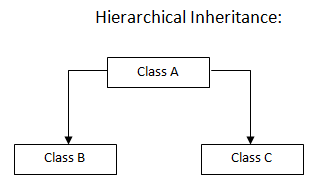C++ Program for Hierarchical Inheritance:

```</pre>
#include <iostream>
using namespace std;
class A
{
public :
int a,b;
void getdata()
{
cout<<"Enter the two operands: "<<endl;
cin>>a>>b;
}
};

class B : public A
{
public:
int x,y;
void campare()
{
if(x>y)
{
cout<<"First operand is greater than the second one."<<endl;
}
else
{
cout<<"Second operand is greater than the first one."<<endl;
}
}
};

class C : public A
{
public:
int p,q;
void calculate()
{
if(p%q==0)
{
cout<<"First operand is divisible by the second one."<<endl;
}
else
{
cout<<"First operand is not divisible by the second one."<<endl;
}
}
};

int main()
{
B obj_1;
obj_1.getdata();
obj_1.a= obj_1.x;
obj_1.b= obj_1.y;
obj_1.campare();

C obj_2;
obj_2.getdata();
obj_2.a= obj_2.p;
obj_2.b= obj_2.q;
obj_2.calculate();
return 0;
}
<pre>```

Output:

```Enter the two operands: 23 31
Second operand is greater than the first one.
Enter the two operands: 42 21
First operand is divisible by the second one.```

#### 5. Hybrid Inheritance:

When a combination of the above-mentioned types is used then it is called hybrid inheritance.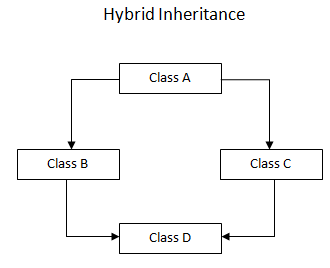As seen in the diagram above,

• Class B and class C are inherited from class A.
• Class D is inherited from two class B and class C.

Indirectly class D is inherited from class A, twice, through class B and class C.

Hence, class D will have two sets of copies of data members of class A. One through class B and the other through class C.

This creates programming ambiguity and should be avoided. For this, you need to declare base class as a virtual base class.

What is the virtual base class?

It can be avoided by making a common base class (ancestor class) as a ‘virtual base class’ while declaring the direct or intermediate base classes.

Example:

```class A
{
Body of class A
};
class B : virtual public A
{
Body of class B
};

class C : virtual public A
{
Body of class C
};
class D : public B, public C
{
Body of class C
};```

When class is made virtual base class then special care is taken by c++ to make sure that only one copy of that class is inherited regardless of how many inheritance paths exists between the virtual base class and derived class.

### Conclusion:

Inheritance is one of the very important concepts in C++ object-oriented programming. You will find many use case scenarios of inheritance while working on your real project.

Many get confused over multiple and multilevel inheritances. Hope after going through this tutorial you have cleared all your doubts.

This is all about different types of inheritance in C++. If you have any further question, please write in the comment section below.